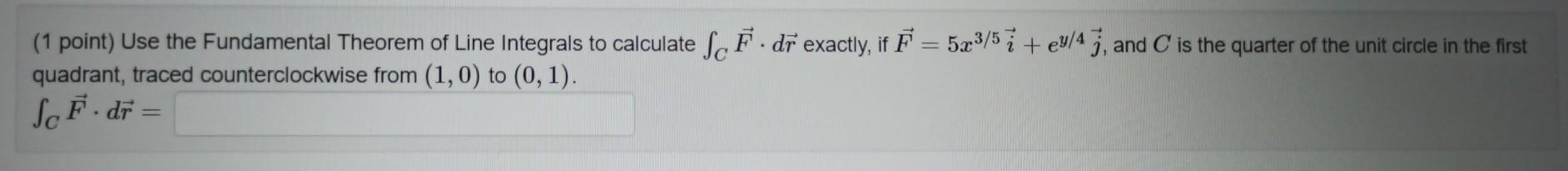### Create an Account

Already have account?

### Forgot Your Password ?

Home / Questions / (1 point) Use the Fundamental Theorem of Line Integrals to calculate Sc F. dr exactly, if ...

# (1 point) Use the Fundamental Theorem of Line Integrals to calculate Sc F. dr exactly, if F = 523/57 + ey/47, and C is the quarter of the unit circle in the first quadrant, traced counterclockwise fro

(1 point) Use the Fundamental Theorem of Line Integrals to calculate Sc F. dr exactly, if F = 523/57 + ey/47, and C is the quarter of the unit circle in the first quadrant, traced counterclockwise from (1,0) to (0,1). Sot dr =Apr 15 2021 View more View Less

#### Answer (Solved)Subscribe To Get Solution